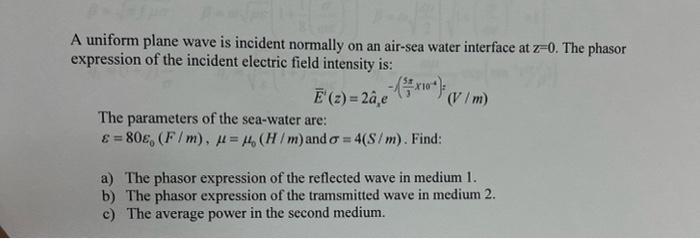# (Solved): please hurry i only have 30 mins. A uniform plane wave is incident normally on an air-sea water inte ...

please hurry i only have 30 mins.A uniform plane wave is incident normally on an air-sea water interface at $$\mathrm{z}=0$$. The phasor expression of the incident electric field intensity is: $\bar{E}^{\prime}(z)=2 \hat{a}_{x} e^{-\left(\frac{5 \pi}{3} \times 10^{4}\right):}(V / m)$ The parameters of the sea-water are: $$\varepsilon=80 \varepsilon_{0}(F / m), \mu=\mu_{0}(H / m)$$ and $$\sigma=4(S / m)$$. Find: a) The phasor expression of the reflected wave in medium 1 . b) The phasor expression of the tramsmitted wave in medium 2 . c) The average power in the second medium.

We have an Answer from Expert